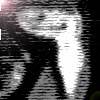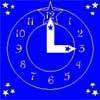#### You may also like### Clock Hands

This investigation explores using different shapes as the hands of the clock. What things occur as the the hands move.### Watch the Clock

During the third hour after midnight the hands on a clock point in the same direction (so one hand is over the top of the other). At what time, to the nearest second, does this happen?### Estimating Angles

How good are you at estimating angles?

# Take the Right Angle

## Take the Right Angle

 How many times in twelve hours do the hands of a clock form a right angle? Use the + and - buttons below to move the hands of the NRICH clock, then click on the right angle symbol to check your answer. Full Screen Version What are these times to the nearest minute?

### Why do this problem?

This problem is one which could be done as an introduction when extending or revising work on time and clocks. It will encourage the use of digital times.

### Key questions

At what time is the first right angle after $12$ o'clock?

Why is it not just $15$ minutes later?

Can you write down these times using digital notation?

Why is the number of times not divisible by $4$?

### Possible extension

Learners could find out the times for $24$ hours and record using $24$ hour digital notation.

### Possible support

Suggest counting round a clock, or using the interactive clock in the question.